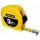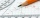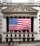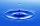# Fraction calculator

The calculator performs basic and advanced operations with fractions, expressions with fractions combined with integers, decimals, and mixed numbers. It also shows detailed step-by-step information about the fraction calculation procedure. Solve problems with two, three, or more fractions and numbers in one expression.

## Result:

### 41/2 + 13/5 = 61/10 = 6 1/10 = 6.1

Spelled result in words is sixty-one tenths (or six and one tenth).

### How do you solve fractions step by step?

1. Conversion a mixed number 4 1/2 to a improper fraction: 4 1/2 = 4 1/2 = 4 · 2 + 1/2 = 8 + 1/2 = 9/2

To find a new numerator:
a) Multiply the whole number 4 by the denominator 2. Whole number 4 equally 4 * 2/2 = 8/2
b) Add the answer from previous step 8 to the numerator 1. New numerator is 8 + 1 = 9
c) Write a previous answer (new numerator 9) over the denominator 2.

Four and one half is nine halfs
2. Conversion a mixed number 1 3/5 to a improper fraction: 1 3/5 = 1 3/5 = 1 · 5 + 3/5 = 5 + 3/5 = 8/5

To find a new numerator:
a) Multiply the whole number 1 by the denominator 5. Whole number 1 equally 1 * 5/5 = 5/5
b) Add the answer from previous step 5 to the numerator 3. New numerator is 5 + 3 = 8
c) Write a previous answer (new numerator 8) over the denominator 5.

One and three fifths is eight fifths
3. Add: 9/2 + 8/5 = 9 · 5/2 · 5 + 8 · 2/5 · 2 = 45/10 + 16/10 = 45 + 16/10 = 61/10
For adding, subtracting, and comparing fractions, it is suitable to adjust both fractions to a common (equal, identical) denominator. The common denominator you can calculate as the least common multiple of both denominators - LCM(2, 5) = 10. In practice, it is enough to find the common denominator (not necessarily the lowest) by multiplying the denominators: 2 × 5 = 10. In the following intermediate step, the fraction result cannot be further simplified by canceling.
In other words - nine halfs plus eight fifths = sixty-one tenths.

#### Rules for expressions with fractions:

Fractions - simply use a forward slash between the numerator and denominator, i.e., for five-hundredths, enter 5/100. If you are using mixed numbers, be sure to leave a single space between the whole and fraction part.
The slash separates the numerator (number above a fraction line) and denominator (number below).

Mixed numerals (mixed fractions or mixed numbers) write as integer separated by one space and fraction i.e., 1 2/3 (having the same sign). An example of a negative mixed fraction: -5 1/2.
Because slash is both signs for fraction line and division, we recommended use colon (:) as the operator of division fractions i.e., 1/2 : 3.

Decimals (decimal numbers) enter with a decimal point . and they are automatically converted to fractions - i.e. 1.45.

The colon : and slash / is the symbol of division. Can be used to divide mixed numbers 1 2/3 : 4 3/8 or can be used for write complex fractions i.e. 1/2 : 1/3.
An asterisk * or × is the symbol for multiplication.
Plus + is addition, minus sign - is subtraction and ()[] is mathematical parentheses.
The exponentiation/power symbol is ^ - for example: (7/8-4/5)^2 = (7/8-4/5)2

#### Examples:

subtracting fractions: 2/3 - 1/2
multiplying fractions: 7/8 * 3/9
dividing Fractions: 1/2 : 3/4
exponentiation of fraction: 3/5^3
fractional exponents: 16 ^ 1/2
adding fractions and mixed numbers: 8/5 + 6 2/7
dividing integer and fraction: 5 ÷ 1/2
complex fractions: 5/8 : 2 2/3
decimal to fraction: 0.625
Fraction to Decimal: 1/4
Fraction to Percent: 1/8 %
comparing fractions: 1/4 2/3
multiplying a fraction by a whole number: 6 * 3/4
square root of a fraction: sqrt(1/16)
reducing or simplifying the fraction (simplification) - dividing the numerator and denominator of a fraction by the same non-zero number - equivalent fraction: 4/22
expression with brackets: 1/3 * (1/2 - 3 3/8)
compound fraction: 3/4 of 5/7
fractions multiple: 2/3 of 3/5
divide to find the quotient: 3/5 ÷ 2/3

The calculator follows well-known rules for order of operations. The most common mnemonics for remembering this order of operations are:
PEMDAS - Parentheses, Exponents, Multiplication, Division, Addition, Subtraction.
BEDMAS - Brackets, Exponents, Division, Multiplication, Addition, Subtraction
BODMAS - Brackets, Of or Order, Division, Multiplication, Addition, Subtraction.
GEMDAS - Grouping Symbols - brackets (){}, Exponents, Multiplication, Division, Addition, Subtraction.
Be careful, always do multiplication and division before addition and subtraction. Some operators (+ and -) and (* and /) has the same priority and then must evaluate from left to right.

## Fractions in word problems:

• Evaluate expressionEvaluate expression using BODMAS rule: 1 1/4+1 1/5÷3/5-5/8
• Food weightStacie is a resident at the medical facility where you work. You are asked to chart the amount of solid food that she consumes. For the noon meal, today she ate 1/2 of a 3-ounce serving of meatloaf, 3/4 of her 3-ounce serving of mashed potatoes, and 1/3 o
• Sum three fractionsWork out the sum of 1/4, 1/5 and 3/10.
• Conversion of unitsComplete the following length data
• The book 4Mr. Kinion read 3 3/4 chapters in his book on Monday. He then read 2 4/6 more chapters on Tuesday. How many chapters has he read so far?Why does 1 3/4 + 2 9/10 equal 4.65? How do you solve this?What is 4 1/2+2/7-213/14?Calculate the sum of 1/5 of a right angle and 3/4 of a right angle and 3/4 of a straight angleFor the week of July 22, the following day to day changes in the stock market was recorded a certain stock: -2 on Monday; +4 Tuesday; -8 Wednesday; + 2 1/2 Thursday; - 3 1/4 Friday. The stock began the week at 78 points. How many points did it finish withDolor puts 3 1/2 pails of water into a water container in the bathroom every day. Her daughter, Lei, uses 2 1/4 pails of water every day when taking a bath. If the water container had 5 5/8 pails of water at the start, how much water is left in it after 5During a visit to an orchard, Greg picked 3/5 of a bag of delicious golden apples, 4/5 of a bag of Macintosh apples, 2/5 of a bag of Cortland apples, 1/5 of a bag of Bartlett pears, and 4/5 of a bag of Bosch pears. How many bags of fruit to Greg pick in tAdd this two mixed numbers: 1 5/6 + 2 2/11=Evaluate 2x+6y when x=- 4/5 and y=1/3. Write your answer as a fraction or mixed number in simplest form.